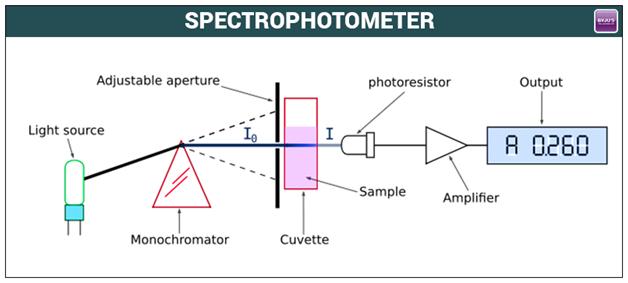# Spectrophotometer Principle

The spectrophotometer is an instrument which measures the amount of light that a sample absorbs. The spectrophotometer works by passing a light beam through a sample to measure the light intensity of a sample. These instruments are used in the process of measuring colour and used for monitoring colour accuracy throughout production. They are primarily used by researchers and manufacturers everywhere. The major Spectrophotometer Applications are limitless as they are used in practically every industrial and commercial field. However, it finds its major applications in liquids, plastics, paper, metals and fabrics. This helps in ensuring that the colour chosen remains consistent from its original conception to the final, finished product.

## Spectrophotometer InstrumentationA spectrophotometer is made up of two instruments: a spectrometer and a photometer. The spectrometer is to produce light of any wavelength, while the photometer is to measure the intensity of light. The spectrophotometer is designed in a way that the liquid or a sample is placed between spectrometer and photometer. The photometer measures the amount of light that passes through the sample and delivers a voltage signal to the display. If the absorbing of light changes, the voltage signal also changes. Spectrophotometers come in a variety of shapes and sizes and have multipurpose uses to them. The different types of spectrophotometers available are all different from one another, based on their application and desired functionality. The most popular spectrophotometers are 45 degrees, sphere and multi-angle spectrophotometers. Another closely related concept is Spectroscopy, that simply measures the absorption of light from its source and the intensity of light as well.

The basic spectrophotometer instrument consists of a light source, a digital display, a monochromator, a wavelength sector to transmit a selected wavelength, a collimator for straight light beam transmission, photoelectric detector and a cuvette to place a sample.

The intensity of light is symbolized as l0 measure the number of photons per second. When the light is passed through the blank solution, it does not absorb light and is symbolized as (l). Other important factors are Absorbance (A) and Transmittance (T).

$$\begin{array}{l}T = \frac{l}{l_{0}}\end{array}$$

$$\begin{array}{l}A = -log_{10} \ T\end{array}$$

Here, we need to measure the intensity of light that passes a blank solution, and later measure the intensity of light passing a sample. Calculate the transmittance and the absorbance. For the measurement of absorbance, we can use an isosbestic point where the absorbance and wavelength of two or more species are the same.

The number of protons transmit and absorb totally depending on the length of the cuvette and the concentration of the sample.

The transmittance and absorption relation is:

$$\begin{array}{l}Absorbance \ (A) = -log (T) = – log(T) = -log\left ( \frac{l_{t}}{l_{0}} \right )\end{array}$$

The transmittance of an unknown sample can be calculated using the formula given below.

$$\begin{array}{l}Transmittance \ (T) = \frac{l_{t}}{l_{o}}\end{array}$$

Here,

$$\begin{array}{l}l_{t}= Light\ intensity\ after\ passing\ via\ cuvette\end{array}$$
$$\begin{array}{l}{l_{0}}= Light\ intensity\ before\ passing\ via\ cuvette\end{array}$$

Further, there are several varieties of spectrophotometer devices such as UV Spectrometry, atomic emission spectrophotometry and atomic absorption spectrophotometry and much more. It can also be classified into two types based on the range of light source wavelengths like IR spectrophotometer and UV-visible spectrophotometer. Some major real-life applications of spectrophotometry in various fields are laundry soap, carpeting and production of small parts such as toys or intricate machinery. The major types of spectrophotometers are categorized into 2, these are mainly portable spectrophotometers and bench spectrophotometers, they both are unique and have their own uses.

## Frequently Asked Questions – FAQs

Q1

### What is spectrophotometer used for?

A spectrophotometer is an analytical instrument used for the objective calculation of visible light, UV light, or infrared light emission or reflection. Spectrophotometers measure intensity as a function of the wavelength of the light source.

Q2

### What is the basic principle of spectrophotometer?

Spectrophotometry is a procedure for determining how much light is reflected by a chemical material by measuring the strength of light as a light beam travels through the sample solution. The fundamental theory is that light is absorbed or emitted over a certain wavelength spectrum by each compound.

Q3

### What is the difference between a spectrometer and a spectrophotometer?

A spectrometer is an aspect of the most responsible spectrophotometer for the calculation of different objects. A spectrophotometer is a comprehensive device that involves a light source, a way of collecting the light that has interacted with the objects being measured, and a measurement spectrometer.

Q4

### How does a spectrophotometer work?

The source of light is given by a lamp. The light beam strikes the diffraction grating, which acts like a mirror and divides the light into the wavelengths of its elements. The grating is rotated such that the exit slit is only penetrated by a single wavelength of light. Then with the sample, the light interacts.

Q5

### What is a blank in spectrophotometry?

A blank is a sample containing everything except for the significance analyte. For example, if you are conducting an experiment with UV-Vis to measure Green Fluorescent Protein concentrations, the protein must be dissolved in a solvent. The blank is a sample of the solvent itself.

Take up a quiz on spectrophotometer-principle